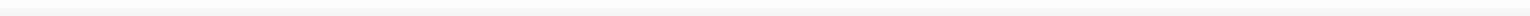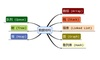• 表达式求值数据结构课程设计，最近编写的，适用于学生
• 后缀表达式求值栈的最一个应用例子，计算一个后缀表达式的值。这个例子中仍然用栈的数据结构。不过，当扫描表达式的时候，这次是操作数压栈等待，不是转换算法中那样让操作符等待。另一条思路是，无论何时看到输入一...
从本节开始，删除原版的英文，直接发译后的文稿。后缀表达式求值栈的最一个应用例子，计算一个后缀表达式的值。这个例子中仍然用栈的数据结构。不过，当扫描表达式的时候，这次是操作数压栈等待，不是转换算法中那样让操作符等待。另一条思路是，无论何时看到输入一个操作符，最近的两个操作数就是操作对象。为了说清楚一点，考虑表达式 4 5 6 * +。从左到右扫描时，首先得到4和5，不过此时，并不知道怎样处理这两个数，直到看到后面的操作符。所以要把这两个数先压栈，得到操作符以后再出栈。这个例子中，下一个符号仍然是操作数，所以照旧压栈，并检查下一个。现在看到操作符*，这意味着最近两个操作数要用来做乘法。出栈两次，得到两个操作数并相乘(在本例中是结果是30)这个计算结果要压回到栈内，并作为下一个操作符的对象。当最后一个操作符工作结束，栈内应该只有一个数值，出栈并作为计算结果返回。图10显示了这个求值过程中，栈内容的变化。图11显示了一个稍微复杂的表达式求值过程。 7 8 + 3 2 + /。这个例子中有两点要注意。第一，栈的大小，随着子表达式的计算过程而膨胀，收缩，再膨胀。第二，除法操作符要小心处理，因为后缀表达式的操作数顺序不变，但当两个操作数出栈时，顺序反了。因为除法不支持交换律，所以15/5与5/15不同，必须保证顺序没有交错。喎�"https://www.2cto.com/kf/ware/vc/" target="_blank" class="keylink">vcD4KPGltZyBzcmM9"https://www.2cto.com/uploadfile/Collfiles/20140317/20140317090702245.jpg" alt="\">算法假定后缀表达式是一系列被空格分隔的字符，操作符是 * /+ -，操作数假定是一位整数。最终结果也是整数。1建立一个空栈，operandStack2字符串使用split转为列表3从左到右检索列表如果是操作数，字符转为整数，压栈如果是操作符，出栈两次。第一次出栈的是第二个操作数，第二次出栈的是第一个操作数。计算结果，并压回栈。4检索结束，出栈结果就是返回值。完整的函数代码如下，其中的doMath是算法辅助函数，定义为两个操作数和一个操作符的计算。from pythonds.basic.stack import Stackdef postfixEval(postfixExpr):operandStack = Stack()tokenList =postfixExpr.split()for token in tokenList:if token in"0123456789":operandStack.push(int(token))else:operand2 =operandStack.pop()operand1 =operandStack.pop()result =doMath(token,operand1,operand2)operandStack.push(result)return operandStack.pop()def doMath(op, op1, op2):if op == "*":return op1 * op2elif op == "/":return op1 / op2elif op == "+":return op1 + op2else:return op1 - op2print(postfixEval('7 8 + 3 2 + /'))
展开全文• 在文章数据结构入门(一)栈的实现中，我们已经知道了如何去实现“栈”这个数据结构，并且介绍了一个它的应用：数的进制转换。在本文中，将会介绍栈的第二个应用，也就是栈在数学表达式求值中的应用。我们分以下几步对...
在文章数据结构入门(一)栈的实现中，我们已经知道了如何去实现“栈”这个数据结构，并且介绍了一个它的应用：数的进制转换。在本文中，将会介绍栈的第二个应用，也就是栈在数学表达式求值中的应用。我们分以下几步对数学表达式进行求值。栈的实现；中缀表达式转后缀表达式；后缀表达式求值。先不着急明白上述术语，你看下去就会明白了。栈的实现以下是栈的Python实现(Stack.py)，代码如下：# -*- coding: utf-8 -*-class Empty(Exception):# Error attempting to access an element from an empty containerpassclass Stack:# initializedef __init__(self):self.__data = []# length of Stackdef __len__(self):return len(self.__data)# whether the Stack is emptydef is_empty(self):return len(self.__data) == 0# push an element is Stackdef push(self, e):self.__data.append(e)# top element of Stackdef top(self):if self.is_empty():raise Empty('Stack is empty')return self.__data[-1]# remove the top element of Stackdef pop(self):if self.is_empty():raise Empty('Stack is empty')return self.__data.pop()# clear the Stackdef clear(self):while not self.is_empty():self.pop()中缀表达式转后缀表达式首先，我们来看一下数学表达式的三种形式：前缀表达式，中缀表达式，后缀表达式。中缀表达式(Infix Expression)就是我们平时常用的书写方式，带有括号。前缀表达式(Prefix Expression)要求运算符出现在运算数字的前面，后缀表达式(Postfix Expression)要求运算符出现在运算数字的后面，一般这两种表达式不出现括号。示例如下：中缀表达式前缀表达式后缀表达式A + B * C + D+ + A * B C DA B C * + D +(A + B) * (C + D)* + A B + C DA B + C D + *A * B + C * D+ * A B * C DA B * C D * +A + B + C + D+ + + A B C DA B + C + D +一般在计算机中，为了方便对表达式求值，我们需要将熟悉的中缀表达式转化为后缀表达式。中缀表达式转后缀表达式的算法如下：创建一个空栈opstack，用于储存运算符。创建一个空的列表，用于储存输出结果。将输入的中缀表达式(字符串形式)用字符串的split方法转化为一个列表。从左到右对该列表进行遍历操作(元素为token)，如下：如果token为运算数，则将它添加(append)至输出列表中。如果token为左小括号，则将它压入(psuh)到opstack中。如果token是右小括号，则对opstack进行pop操作，直至对应的左小括号被移出。将移出的运算符添加(append)到输出列表的末端。如果token是 *, /, +, -, 中的一个，则将其压入(push)到opstack中。注意，先要移除那些运算优先级大于等于该token的运算符，并将它们添加到输出列表中。当上述过程结果后，检查opstack。任何还在opstack中的运算符都应移除，并将移出的运算符添加(append)到输出列表的末端。上述过程的完整Python代码如下：# -*- coding: utf-8 -*-from Stack import Stack# 中缀表达式转化为后缀表达式def infixToPostfix(infixexpr):prec = {"*": 3, "/": 3, "+": 2, "-": 2, "(": 1}opStack = Stack()postfixList = []tokenList = infixexpr.split()for token in tokenList:if token.isdigit() or '.' in token:postfixList.append(token)elif token == '(':opStack.push(token)elif token == ')':topToken = opStack.pop()while topToken != '(':postfixList.append(topToken)topToken = opStack.pop()else:while (not opStack.is_empty()) and (prec[opStack.top()] >= prec[token]):postfixList.append(opStack.pop())opStack.push(token)while not opStack.is_empty():postfixList.append(opStack.pop())return " ".join(postfixList)# inExpr = "( ( 1 + 2 ) * 3 ) * ( 3 - 1.2 )"inExpr = "10 + 3 * 5 / ( 16 - 4 )"postExpr = infixToPostfix(inExpr)print(postExpr)输出结果如下：10 3 5 * 16 4 - / +后缀表达式求值当把中缀表达式转化为后缀表达式之后，我们再利用栈对后缀表达式求值。其具体的算法如下：建立一个栈来存储待计算的运算数；遍历字符串，遇到运算数则压入栈中，遇到运算符则出栈运算数(2次)，进行相应的计算，计算结果是新的操作数，压入栈中，等待计算；按上述过程，遍历完整个表达式，栈中只剩下最终结果；完整的Python代码如下：(接以上代码)# -*- coding: utf-8 -*-from Stack import Stack# 两个数的运算, 除法时分母不为0def operate(op, num1, num2):if num2 == 0:raise ZeroDivisionErrorelse:res = {'+': num1 + num2,'-': num1 - num2,'*': num1 * num2,'/': num1 / num2,}return res[op]# 将字符串转化为浮点型或整型数字def str2num(s):if '.' in s:return float(s)else:return int(s)# 后缀表达式求值def evalPostfix(e):tokens = e.split()  # 后缀表达式转化为列表s = Stack()for token in tokens:if token.isdigit() or '.' in token:  # 如果当前元素是数字s.push(str2num(token))elif token in '+-*/':  # 如果当前元素是运算符op2 = s.pop()op1 = s.pop()s.push(operate(token, op1, op2))  # 计算结果入栈return s.pop()# inExpr = "( ( 1 + 2 ) * 3 ) * ( 3 - 1.2 )"inExpr = "10 + 3 * 5 / ( 16 - 4 )"postExpr = infixToPostfix(inExpr)print(postExpr)result = evalPostfix(postExpr)print(result)输出结果：11.25请务必注意，我们输入的中缀表达式中，每个运算符或运算符要用空格隔开。参考文献注意：本人现已开通微信公众号： Python爬虫与算法(微信号为：easy_web_scrape)， 欢迎大家关注哦~~
展开全文• 表达式求值 Java代码 /** * 整数表达式运算 */ public class EvaluateExpression { /** * 表达式 */ private String expression; /** * 最初的表达式 */ private String initExpression; /** * 运算符栈 */ private...
展开全部1. 定义优先级和优先级表Java代码/*** 运算符优先权*/public enum Precede {/*** 优先权高*/LARGER,/*** 优先权低*/LESS;/*** 优先级表*      +   -   *   /*  +   >    >    <    <*  -   >    >    <    <*  *   >    >    >    >*  /   >    >    >    >*/private static Precede[][] precedes = new Precede;static {// 根据优先级表初始化precedes数组for (int i = 0; i < precedes.length; i++) {for (int j = 0; j < precedes[i].length; j++) {if ((i == 0 || i == 1) && j > 1) {precedes[i][j] = LESS;} else {precedes[i][j] = LARGER;}}}}/*** 判断32313133353236313431303231363533e58685e5aeb9313333396632652个运算符的优先级*/public static Precede judgePrecede(char operand1, char operand2) {int left = getIndex(operand1);int right = getIndex(operand2);return precedes[left][right];}/*** 获取运算符对应的数组索引*/private static int getIndex(char operand) {switch (operand) {case '+':return 0;case '-':return 1;case '*':return 2;case '/':return 3;default:throw new IllegalArgumentException();}}}2. 表达式求值Java代码/*** 整数表达式运算*/public class EvaluateExpression {/*** 表达式*/private String expression;/*** 最初的表达式*/private String initExpression;/*** 运算符栈*/private MyStack optr = new MyArrayStack();/*** 操作数栈*/private MyStack opnd = new MyArrayStack();/*** 表明下一个是否应该是数字*/private boolean numberNext;public EvaluateExpression(String expression) {this.expression = expression;this.initExpression = expression;numberNext = true;}/*** 求值*/public Integer evaluate() {delBlank();handleParentheses();while (true) {if ("".equals(expression)) {break;}if (expression.matches("^-?\\d+.*\$") && numberNext) {opnd.push(getInteger());continue;} else {Character operand = expression.charAt(0);numberNext = true;expression = expression.substring(1);Character pop = optr.pop();if (pop == null) {optr.push(operand);continue;} else {Precede precede = Precede.judgePrecede(pop, operand);switch (precede) {// 优先级高时运算前2个操作数case LARGER: {optr.push(operand);Integer next = opnd.pop();Integer last = opnd.pop();evaluateNow(last, pop, next);break;}// 优先级低时运算前一个操作数和后一个操作数case LESS: {optr.push(pop);Integer last = opnd.pop();Integer next = getInteger();evaluateNow(last, operand, next);break;}}}}}// 运算结果Integer result = null;if (optr.length() == 0 && opnd.length() == 1) {result = opnd.pop();} else if (optr.length() == 1 && opnd.length() == 2) {Integer next = opnd.pop();Integer last = opnd.pop();evaluateNow(last, optr.pop(), next);result = opnd.pop();} else {throw new RuntimeException();}return result;}/*** 进行实际的运算，并将结果入栈*/private void evaluateNow(Integer last, Character operand, Integer next) {switch (operand) {case '+':opnd.push(last + next);break;case '-':opnd.push(last - next);break;case '*':opnd.push(last * next);break;case '/':opnd.push(last / next);break;}}/*** 获得表达式开头部分的整数*/private Integer getInteger() {StringBuilder sb = new StringBuilder();int count = 0; // 整数位boolean lessZero = false; // 是否是负数if (expression.startsWith("-")) {sb.append("-");count++;lessZero = true;}int i = (lessZero ? 1 : 0);for (; i < expression.length(); i++) {char c = expression.charAt(i);if (c >= '0' && c <= '9') {sb.append(c);count++;} else {break;}}expression = expression.substring(count);numberNext = false;return Integer.valueOf(sb.toString());}/*** 处理括号. 将括号内的字符串作为子表达式计算.*/private void handleParentheses() {while (expression.contains("(")) {// 左括号的索引int left = 0;// 右括号的索引int right = 0;// 左括号的数量int count = 0;// 求出左括号索引left = expression.indexOf('(');// 求出对应的右括号索引for (int i = left; i < expression.length(); i++) {char c = expression.charAt(i);if (c == ')') {count--;// count为0时才是对应的右括号if (count == 0) {right = i;break;}} else if (c == '(') {count++;} else {continue;}}// 左右括号之间是一个子表达式, 计算子表达式的值，并根据结果构造出新的表达式EvaluateExpression evaluateExpression = new EvaluateExpression(expression.substring(left + 1, right));expression = expression.substring(0, left) + evaluateExpression.evaluate()+ expression.substring(right + 1);}}/*** 删除表达式中的空白字符*/private void delBlank() {expression = expression.replaceAll("\\s", "");}@Overridepublic String toString() {return initExpression;}}3. 进行测试Java代码@Testpublic void testEvaluate() {EvaluateExpression expression = new EvaluateExpression("1 + 2 ");System.out.println(expression + " = " + expression.evaluate());expression = new EvaluateExpression("4 + 2 * 3 - 10 / 5");System.out.println(expression + " = " + expression.evaluate());expression = new EvaluateExpression("(1+2) * (4 + 5) - (9 / 7)");System.out.println(expression + " = " + expression.evaluate());expression = new EvaluateExpression("(1 + (3 * (4 - 9)))");System.out.println(expression + " = " + expression.evaluate());expression = new EvaluateExpression("(1 + (3 * (4 - 9))) + (3 * (2 + 3))");System.out.println(expression + " = " + expression.evaluate());}测试的结果为:1 + 2  = 34 + 2 * 3 - 10 / 5 = 8(1+2) * (4 + 5) - (9 / 7) = 26(1 + (3 * (4 - 9))) = -14(1 + (3 * (4 - 9))) + (3 * (2 + 3)) = 1本回答由提问者推荐已赞过已踩过<你对这个回答的评价是？评论收起
展开全文• /*8588 表达式求值 时间限制:1000MS 代码长度限制:10KB 提交次数:3462 通过次数:1255 题型: 编程题 语言: G++;GCC Description 顺序栈的基本操作如下： 利用栈编写表达式求值程序：输入含有“+”、“-”、“*”、...
/*8588 表达式求值
时间限制:1000MS  代码长度限制:10KB
提交次数:3462 通过次数:1255

题型: 编程题   语言: G++;GCC
Description
顺序栈的基本操作如下：
利用栈编写表达式求值程序：输入含有“+”、“-”、“*”、“/”四则运算的表达式，其中负数要用（0-正数）表示，并以=结束。要求输出表达式的值（两运算符号的优先关系见教材表3.1）。
输入格式
第一行：一个算术表达式
输出格式
第一行：算术表达式的值
输入样例
3*(9-7)=
输出样例
6

若 ch 是数字，直接压入操作数栈OPND；
若 ch 是'('，直接入栈OPTR;若 ch 是')'，若OPTR 和 OPND 非空，弹出OPTR的栈顶操作符，弹出OPND栈顶的两个操作数，做运算，然后见个结果压入栈OPND，直到弹出的OPTR栈顶元素时')';
若 ch 是操作符(比如+, -, *, /)，如果OPTR栈顶元素是 (，直接入栈OPTR，如果不是'('且OPTR栈非空且栈顶元素操作符的优先级大于ch，那么弹出OPTR的栈顶操作符，并弹出OPND中栈顶的两个元素，做运算，将运算结果入栈OPND，此时，重复这一步操作；否则将ch入栈OPTR；
*/
#include<malloc.h>
#include<stdio.h>
#define OK 1
#define ERROR 0
#define STACK_INIT_SIZE 100 // 存储空间初始分配量
#define STACKINCREMENT 10 // 存储空间分配增量
#include <ctype.h>
#include <string.h>
typedef int SElemType; // 定义栈元素类型
typedef int Status; // Status是函数的类型,其值是函数结果状态代码，如OK等

struct SqStack
{
SElemType *base; // 在栈构造之前和销毁之后，base的值为NULL
SElemType *top; // 栈顶指针
int stacksize; // 当前已分配的存储空间，以元素为单位
}; // 顺序栈

Status InitStack(SqStack &S)
{
// 构造一个空栈S，该栈预定义大小为STACK_INIT_SIZE
S.base = (SElemType*)malloc(STACK_INIT_SIZE * sizeof(SElemType));
if (!S.base)
return ERROR;
S.top = S.base;
S.stacksize = STACK_INIT_SIZE;
return OK;
}

Status Push(SqStack &S, SElemType e)
{
// 在栈S中插入元素e为新的栈顶元素
if (S.top - S.base >= S.stacksize)
{
S.base = (SElemType*)realloc(S.base, (S.stacksize + STACKINCREMENT) * sizeof(SElemType));
if (!S.base)
return ERROR;
S.top = S.base + S.stacksize;
S.stacksize += STACKINCREMENT;
}
*S.top++ = e;
return OK;
}

Status Pop(SqStack &S, SElemType &e)
{
// 若栈不空，则删除S的栈顶元素，用e返回其值，并返回OK；否则返回ERROR
if (S.top == S.base)
return ERROR;
e = *--S.top;
return OK;
}

Status GetTop(SqStack S, SElemType &e)
{
// 若栈不空，则用e返回S的栈顶元素，并返回OK；否则返回ERROR
if (S.top == S.base)
return ERROR;
e = *(S.top - 1);
return OK;
}

int StackLength(SqStack S)
{
// 返回栈S的元素个数
int i;
i = S.top - S.base;
return i;
}

Status StackTraverse(SqStack S)
{
// 从栈顶到栈底依次输出栈中的每个元素
SElemType *p = (SElemType *)malloc(sizeof(SElemType));
p = S.top;
if (S.top == S.base)
printf("The Stack is Empty!");
else
{
printf("The Stack is: ");
p--;
while (p >= S.base)
{
printf("%d ", *p);
p--;
}
}
printf("\n");
return OK;
}

int reuslt(int num1, int num2, int optr)
{
switch (optr)
{
case '+':
return num1 + num2;
case '*':
return num1 * num2;
case '/':
return num2 / num1;
case '-':
return num2 - num1;
}
}
int main()
{
SqStack S1;
InitStack(S1);
SqStack S2;
InitStack(S2);
char s;
gets(s);
int topS2;
int topS1 = 0;
int num1, num2;
int optr;

for (int i = 0; s[i] != '='; i++)
{
if (isdigit(s[i]))
{
if (i!=0&&isdigit(s[i - 1])) {
Pop(S1, topS1);
Push(S1, topS1 * 10 + (s[i] - '0')); //这个是为了处理出现连续输入多位数字的情况
}
else
{
Push(S1, (s[i] - '0'));
}
}
else
{

switch (s[i])
{
case '(':
Push(S2, s[i]);
break;
case ')':
if (StackLength(S1) != 0 && StackLength(S2) != 0)
{
for (Pop(S2, optr); optr != '('; Pop(S2, optr))
{
Pop(S1, num1);
Pop(S1, num2);
Push(S1, reuslt(num1, num2, optr));
}
break;

}
case '+':
GetTop(S2, optr);
if (StackLength(S2) == 0 || optr == '('  )
{
Push(S2, s[i]);
break;
}
else if (optr == '*' || optr == '/' || optr == '+'|| optr == '-')
{
Pop(S1, num1);
Pop(S1, num2);
Pop(S2, optr);
Push(S1, reuslt(num1, num2, optr));
Push(S2, '+');//前两个弹出来了 运算符还是要入栈的
break;
}
case '-':
GetTop(S2, optr);
if (StackLength(S2) == 0 || optr == '('  )
{
Push(S2, s[i]);
break;
}
else if (optr == '*' || optr == '/' || optr == '-'|| optr == '+')
{
Pop(S1, num1);
Pop(S1, num2);
Pop(S2, optr);
Push(S1, reuslt(num1, num2, optr));
Push(S2, '-');//前两个弹出来了 运算符还是要入栈的
break;
}
case '*':
GetTop(S2, optr);
if (StackLength(S2) == 0 || optr == '('  || optr == '-'|| optr == '+')
{
Push(S2, s[i]);
break;
}
else if (optr == '*' || optr == '/' )
{
Pop(S1, num1);
Pop(S1, num2);
Pop(S2, optr);
Push(S1, reuslt(num1, num2, optr));
Push(S2, '*');//前两个弹出来了 运算符还是要入栈的
break;
}
break;
case '/':
GetTop(S2, optr);
if (StackLength(S2) == 0 || optr == '('  || optr == '-'|| optr == '+')
{
Push(S2, s[i]);
break;
}
else if (optr == '*' || optr == '/' )
{
Pop(S1, num1);
Pop(S1, num2);
Pop(S2, optr);
Push(S1, reuslt(num1, num2, optr));
Push(S2, '/');//前两个弹出来了 运算符还是要入栈的
break;
}
break;
break;
}

}

}

while (StackLength(S2) != 0)
{
Pop(S1, num1);
Pop(S1, num2);
Pop(S2, optr);
Push(S1, reuslt(num1, num2, optr));
}
int sum = 0;
GetTop(S1, sum);
printf("%d", sum);
}




展开全文• 在对后缀表达式从左到右扫描的过程中，在对后缀表达式从左到右扫描的过程中，所以要暂存操作数， 在碰到操作符的时候， 再将暂存的两个操作数进行实际的计算仍然是栈的特性：操作符只作用于离它最近的两个操作数如...
• #include <iostream> #include<stdio.h> #include<stdlib.h> #include<malloc.h> #include<string.h> using namespace std; #define OK 1 #define ERROR 0 #define OVERFLOW -2 #define STACK_SIZE 100 #define STAC
• 一、数组数组（Array）是一种线性表数据结构。数组用一块连续的内存空间，来存储相同类型的一组数据，最大的特点就是支持随机访问，但插入、删除操作也因此变得比较低效，平均情况时间复杂度为 O(n)。二、链表链表...
• 数据结构是计算机编程中最重要的内容之一，我们经常会看到一个公式，那就是程序=数据结构+算法。从这个公式我们就能够看出来数据结构是多么的重要，要想写出优雅高效的程序，一定离不开良好的数据结构，今天我们就来...
• python 数据结构与算法1 python常见数据结构性能1.1 List1.1.1 安索引取值和赋值1.1.2 列表append和__add__()1.1.3 使用timeit模块测试执行时间1.1.4 List基本操作的大O数量级1.2 Dict1.2.1 dict数据类型2 线性结构 ...
• 算术表达式求值，所属为C语言种数据结构相关实验。 已编程为主，要懂基本的C++编译原理。
• 用栈的结构来解决表达式求值 a.可以完成四则混合运算 b.可以完成实数的四则运算 c.可以检查表达式的输入是否正确 d.演示表达式求值的操作过程
• 输入数据中含有一些表达式（数量≤1000，长度按含有的运算计，运算符≤30），表达式的运算符只含有加、减、乘、除。表达式中每个数的精度范围在double型内，表达式中没有任何其他运算符，没有括号。 Output 对每...
• 用栈实现各种双操作数操作符的运算 主要用c/c++语言实现
• 数据结构与算法，相信上过这门课的仁都知道，他是多磨可爱，确实你多磨练自己，才会感觉他可爱。我之前天真的以为只要课程结课了，我就不用再受到他的折磨，现在看来，还是太年轻啊，T_T，我现在发现，他总是会...
• // 算术表达式求值的算符优先算法。 // 设OPTR和OPND分别为运算符栈和运算数栈，OP为运算符集合。 StackChar OPTR; // 运算符栈，字符元素 StackFloat OPND; // 运算数栈，实数元素 char TempData;//支持...float aop struct 算法
• 二 课程设计 2算术表达式求值 一需求分析 二程序的主要功能 三程序运行平台 四数据结构 五算法及时间复杂度 六测试用例 七程序源代码 三 感想体会与总结 算术表达式求值 一需求分析 一个算术表达式是由操作数 ...
• 数据结构 栈 表达式求值数据结构表达式求值数据结构 栈 表达式求值
• 数据结构栈实现表达式求值数据结构栈实现表达式求值数据结构栈实现表达式求值数据结构栈实现表达式求值
• 数据结构课程设计 北京理工大学珠海学院计算机科学技术学院 第 PAGE 2 页 第 PAGE 1 页 . 教育资料 TOC \o "1-3" \h \z \u 1前 言 1 2概要设计 1 2.1 数据结构设计 1 2.2 算法设计 1 2.3 ADT描述 2 2.4 功能模块分析...
• 表达式求值中缀表达式求值中缀表达式转后缀表达式后缀表达式求值（逆波兰表达式求值）前缀表达式（波兰表达式） 首先，大家可能不知道前缀表达式和中缀表达式，后缀表达式是什么，其有什么区别呢。我先简单介绍一下...java 算法
• 数据结构表达式求值数据结构表达式求值数据结构表达式求值
• 数据结构 表达式求值 数据结构——表达式求值（堆栈） (参看：严蔚敏《数据结构与算法》) 限于二元运算符的表达式定义： 表达式：：＝（操作数）+（运算符）+（操作数） 操作数：：＝简单变量|表达式 简单变量...
• 唉，刚刚用C++又又一次写了一个较完好的表达式求值程序，最后精简后程序还不到100行。这不经让我想到了大一上学期刚学c语言时自己费了好大的劲，写了几百行并且功能还不是非常齐全(当时还不能计算有括号的表达式)的...
• 算法设计与分析 实验报告 - PAGE 1 - 实验目的 掌握栈后进先出的特点 掌握栈的典型应用后缀表达式求值 实验内容 用键盘输入一个整数后缀表达式操作数的范围是09运算符只含*/而且中间不可以有空格使用循环程序从左向......

# 表达式求值数据结构数据结构 订阅